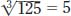# What are a cube and cube root?

Much like a square, a cube is when you multiply a real or complex number by twice itself (making a total of three numbers). Mathematicians express the cube of a number using the superscript 3; or, for example, 23, or 2 × 2 × 2. Unlike a square of a number, the cube of a number will not always be positive, such as -3 × -3 × -3, which equals -9.

A cube root is a number that when multiplied by itself two more times has the product of s, in which t3 = s. For example, the cube root of 125 (s) is 5 (t), or written as; the cube root of -125 is -5.

Close

This is a web preview of the "The Handy Math Answer Book" app. Many features only work on your mobile device. If you like what you see, we hope you will consider buying. Get the App